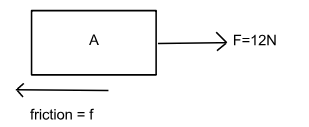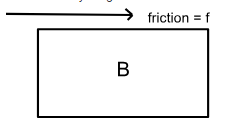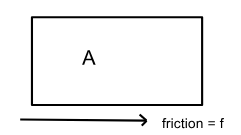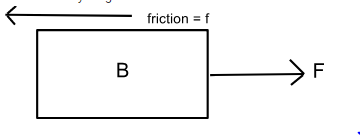#### A block A of mass 4 kg is placed on another block B of mass 5 kg, and the block B rests on a smooth horizontal table. If the minimum force that can be applied on A so that both the blocks move together is 12 N, the maximum force that can be applied on B for the blocks to move together will be :Option: 1 30 NOption: 2 25 NOption: 3 15 NOption: 4 48 NWhen the force of 12 N is applied on block A,

FBD of A isEquation of motion of A is

12 - f = 4a ...(i)

FBD of B isEquation of motion of B is

f = 5a ...(ii)

Dividing (i) and (ii), we get

When force is applied on B:

Frictional force acting between the blocks will remain same.

FBD of A is:Equation of motion of A is

f = 4a ...(iii)

FBD of B isEquation of motion of B is
F - f = 5a ...(iv)

Dividing (iv) by (iii), we get